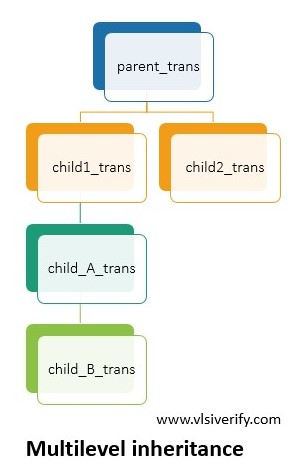Tutorials

An Inheritance is the concept of OOP which allows users to create an extended class from the existing class. The existing class is commonly known as base class or parent class and the newly created extended class is known as a derived class or child class or subclass.

The “extends” keyword is used to inherit the child class from its base class.

1. Child class has access to class properties and class methods of its base class. Thus, inheritance grants re-usability.
2. Along with existing class properties and methods, a derived class can also add new properties and methods based on the requirement.
3. A child class can modify its base class properties and methods without disturbing the base class.
4. Multilevel inheritance is also possible in SystemVerilog. A derived class can also further extended, this is multilevel inheritance.

## Class nomenclature

Parent Class – Base class, Superclass

Child class – Derived class, Subclass, Extended class

### Inheritance Example

A child_trans is an extended class from its parent_trans (base class). A child_class can access its base class properties (data variable) and methods (disp_p function)

``````class parent_trans;
bit [31:0] data;

function void disp_p();
\$display("Value of data = %0h", data);
endfunction
endclass

class child_trans extends parent_trans;
int id;

function void disp_c();
\$display("Value of id = %0h", id);
endfunction
endclass

module class_example;
initial begin
child_trans c_tr;
c_tr = new();
c_tr.data = 5; // child class is updating property of its base class
c_tr.id = 1;

c_tr.disp_p(); // child class is accessing method of its base class
c_tr.disp_c();
end
endmodule``````

Output:

``````Value of data = 5
Value of id = 1``````

## Multilevel inheritance### Example for Multilevel Inheritance​

``````class parent_trans;
bit [31:0] data_p;

function void disp_p();
\$display("parent_trans: Value of data = %0h", data_p);
endfunction
endclass

class child1_trans extends parent_trans;
bit [31:0] data_c1;

function void disp_c1();
\$display("child1_trans: Value of data = %0h", data_c1);
endfunction
endclass

class child2_trans extends parent_trans;
bit [31:0] data_c2;

function void disp_c2();
\$display("child2_trans: Value of data = %0h", data_c2);
endfunction
endclass

class child_A_trans extends child1_trans;
bit [31:0] data_cA;

function void disp_cA();
\$display("child_A_trans: Value of data = %0h", data_cA);
endfunction
endclass

class child_B_trans extends child_A_trans;
bit [31:0] data_cB;

function void disp_cB();
\$display("child1_2_trans: Value of data = %0h", data_cB);
endfunction
endclass

module class_example;
initial begin
child_B_trans cB_tr;
cB_tr = new();
cB_tr.data_p = 2;
cB_tr.data_c1 = 4;
cB_tr.data_cA = 6;
cB_tr.data_cB = 8;
//cB_tr.data_c2 = 3;  // Not possible as child_B_trans is not child class of child2_trans.

cB_tr.disp_p();
cB_tr.disp_c1();
cB_tr.disp_cA();
cB_tr.disp_cB();
end
endmodule``````

Output:

``````parent_trans: Value of data = 2
child1_trans: Value of data = 4
child_A_trans: Value of data = 6
child1_2_trans: Value of data = 8``````

## Overriding base class members

The child class properties and methods override base class properties and methods when both the child and base class have the same naming convention for class properties and method.

``````class parent_trans;
bit [31:0] data = 100;
int id = 1;

function void display();
\$display("Base: Value of data = %0d and id = %0d", data, id);
endfunction
endclass

class child_trans extends parent_trans;
bit [31:0] data = 200;
int id = 2;

function void display();
\$display("Child: Value of data = %0d and id = %0d", data, id);
endfunction
endclass

module class_example;
initial begin
child_trans c_tr;
c_tr = new();

c_tr.display();
end
endmodule``````

Output:

``Child: Value of data = 200 and id = 2``

## Accessing class members in Inheritance

Based on the handle of a class, the corresponding method will be called.

``````class parent_trans;
bit [31:0] data;
int id;

function void display();
\$display("Base: Value of data = %0d and id = %0d", data, id);
endfunction
endclass

class child_trans extends parent_trans;
bit [31:0] data;
int id;

function void display();
\$display("Child: Value of data = %0d and id = %0d", data, id);
endfunction
endclass

module class_example;
initial begin
parent_trans p_tr;
child_trans c_tr;

p_tr = new();
c_tr = new();

p_tr.data = 100;
p_tr.id = 1;

c_tr.data = 200;
c_tr.id = 2;

p_tr.display();
c_tr.display();
end
endmodule``````

Output:

``````Base: Value of data = 100 and id = 1
Child: Value of data = 200 and id = 2``````

System Verilog Tutorials